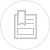# kinetics of the corrosion reactions

The difference between corrosion and immunity can be defined by the electrochemical potential of the corrosion reaction (see above). However, the definition of passivation requires a study of electrochemical reaction kinetics and of the concept of polarisation, i.e. the variation in the electrodes potentials caused by a net current. The relation between the half-cell rate of reaction and its potential is given in the general form :

where, for an electrochemical reaction, E is the potential recorded, E is the thermodynamic potential and the rate of the reaction is defined by the stream of electrons (i) between the cathode and the anode.

An Evans diagram can be used to outline the impact polarisation has on the corrosion process, as seen in figure 2.

The potential (represented by the ordinates axis) is the potential actually applied whereas the current generated by the anodic and cathodic reactions will run in the opposite direction. The Evans diagram represents the absolute value of the half-cell current. Thus, electro-neutrality requirements are met at the point where the anodic and cathodic reaction lines intersect. Potential (Ecorr) and current (Icorr) at this point define the corrosion potential and the current/rate of the overall electrochemical mechanism.

If the cathodic reaction is polarised, the cathodic curve of the Evans diagram will shift towards lower current values with a constant potential b → c. Figure 3 illustrates the net effect.